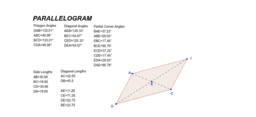pchou

•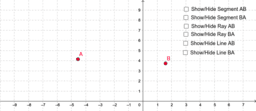Line Segments, Rays, and Lines Definitions

Activity

pchou

•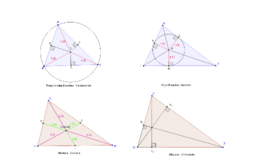Explore circumcenters, incenters, centroids and orthocenters

Activity

pchou

•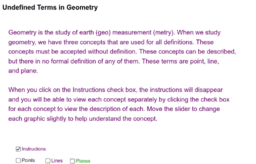Points, Lines, Planes Definitions

Activity

pchou

•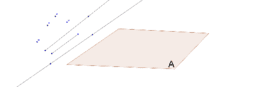Points, Lines, and Planes create

Activity

pchou

•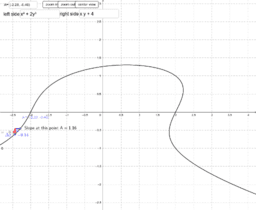Copy of implicit derivatives with graphs

Activity

pchou

•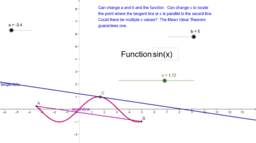Copy of Section 3.2-Mean Value Theorem

Activity

pchou

•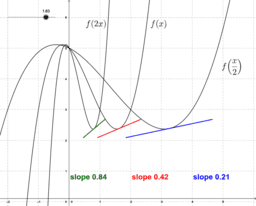Copy of The Chain Rule for Derivatives

Activity

pchou

•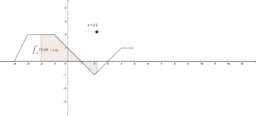Copy of Accumulation functions

Activity

pchou

•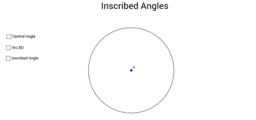Inscribed Angles Central Angles in Circle

Activity

pchou

•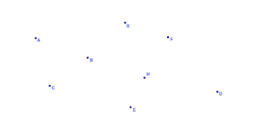Introduction to Geometry

Book

pchou

•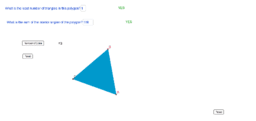Sum of Interior Angles of Polygons

Activity

pchou

•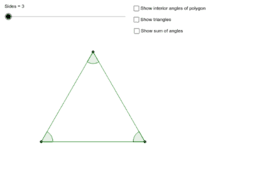Interior Angles of Polygons

Activity

pchou

•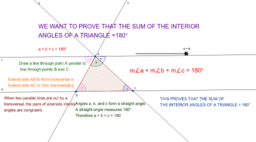Polygon: Interior and Exterior Angles

Book

pchou

•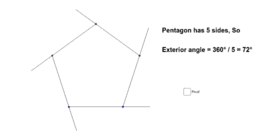Calculating exterior angle of polygon

Activity

pchou

•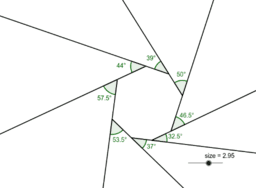Sum of exterior angles of a polygon

Activity

pchou

•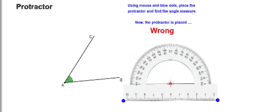Using the protractor

Activity

pchou

•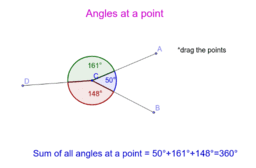Angles 1

Book

pchou

•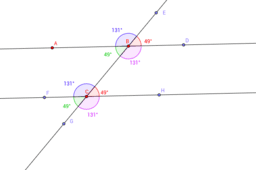Parallel Lines & Transversals

Book

pchou

•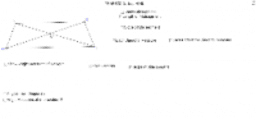Book

pchou

•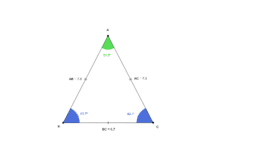Isosceles Triangle

Activity

pchou

•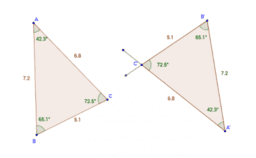Exploring Triangle Congruence

Book

pchou

•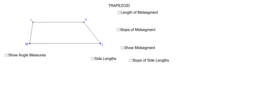Book

pchou

•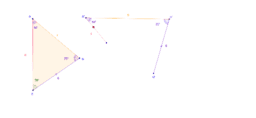More Tests for Congruent Triangles

Book

pchou

•